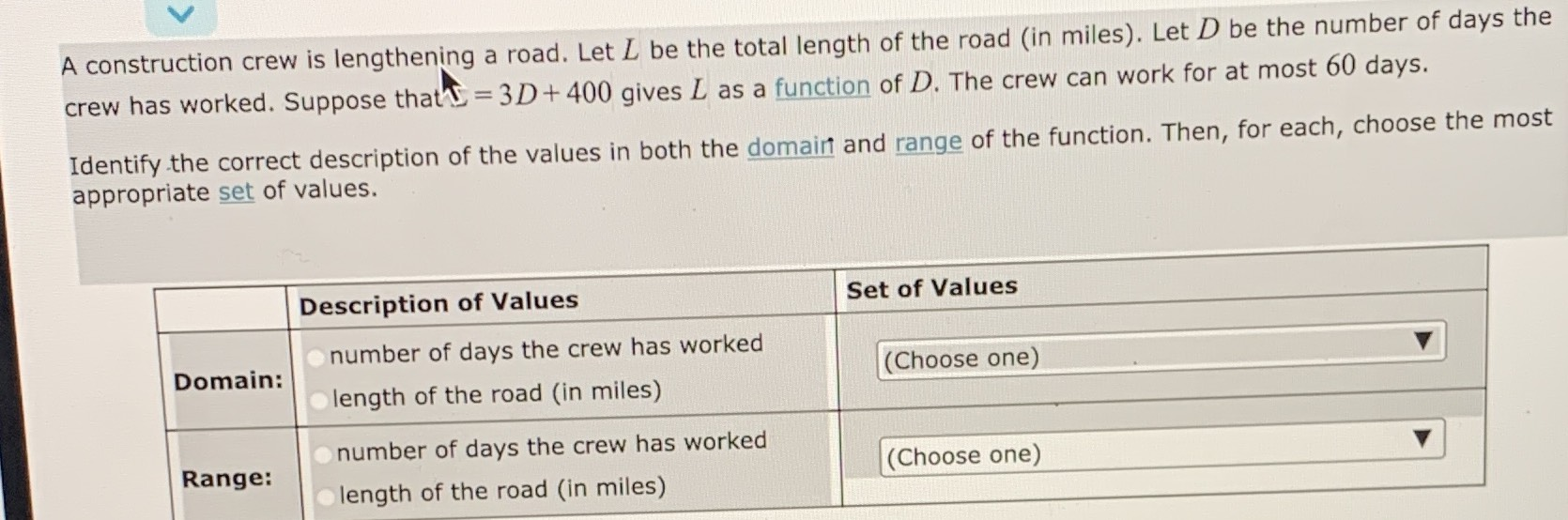### ¿Todavía tienes preguntas de matemáticas?

Pregunte a nuestros tutores expertos
Algebra
PreguntaA construction crew is lengthening a road. Let $$L$$ be the total length of the road (in miles). Let $$D$$ be the number of days the crew has worked. Suppose that $$= 3 D + 400$$ gives $$L$$ as a function of $$D$$ . The crew can work for at most $$60$$ days. Identify the correct description of the values in both the domain and range of the function. Then, for each, choose the most appropriate set of values.